## 细长椭球体在水中自由下落的运动特性实验研究 1)

*西安交通大学航天航空学院机械结构振动与强度国家重点实验室, 西安710049

## EXPERIMENTAL STUDY ON THE KINEMATICS OF FREELY FALLING PROLATE SPHERE IN THE WATER 1)

Zhan Jun*, Zhang Jie*, Yang Juancheng,*,2), Ni Mingjiu*,

*State Key Laboratory for Strength and Vibration of Mechanical Structures, School of Aerospace, Xi'an Jiaotong University, Xi'an 710049, China

School of Engineering Science, University of Chinese Academy of Sciences, Beijing 100049, China

 基金资助: 1)国家自然科学基金.  51636009国家自然科学基金.  51776166国家自然科学基金.  11872296

Received: 2020-01-6   Accepted: 2020-04-21   Online: 2020-05-18Abstract

The motion of solid particles in the liquid is frequently encountered in everyday life and engineering applications. It attracts the devotement of many researchers due to the abundant fluid dynamic phenomena behind the motion of solid particles in the liquid. In the present paper, we experimentally study the falling characteristics of a single prolate sphere particle descending in water under the influence of buoyancy force. The motion tracking platform which consists of two orthogonal high-speed cameras and light sources accompanied by the fluorescence visualization technology is adopted to obtain the paths and vortex structures of the falling prolate sphere particle. The density ratio between the selected prolate sphere and surrounding fluid is 1.2, while the aspect ratio of the selected prolate sphere varies from 2 to 10, the corresponding Archimedes number changes from 400 to 1400, and finally, the terminal Reynolds number is limited in the range from 120 to 1350. During the experiments, we observe five typical types of paths during the falling process of the prolate spheres, which corresponds to small amplitude irregular motion, small-amplitude high-frequency oscillation motion, large-amplitude low-frequency oscillation motion, highly nonlinear motion and rectilinear motion, respectively. And the evolution of the oscillation of velocity and the inclination angle is obtained. Furthermore, we analyze the relationships between the drag coefficient of the freely falling prolate sphere and the Reynolds number during the falling process. Then, by using the fluorescence visualization technique, we identify the different vortex shedding modes, which are responsible for different falling trajectories, and study the influence of vortex shedding on the path of free falling prolate sphere. Finally, comparing with some previous results on the falling characteristics of the slender cylinder, we find the similarities and differences of the motion characteristics between the prolate sphere and the slender cylinder and the potential physical mechanism.

Keywords： wake instability ; prolate sphere ; motion tracking system ; vortex structure

Zhan Jun, Zhang Jie, Yang Juancheng, Ni Mingjiu. EXPERIMENTAL STUDY ON THE KINEMATICS OF FREELY FALLING PROLATE SPHERE IN THE WATER 1). Chinese Journal of Theoretical and Applied Mechanics[J], 2020, 52(3): 716-727 DOI:10.6052/0459-1879-20-005

## 1 实验系统及数据处理方法

### 图1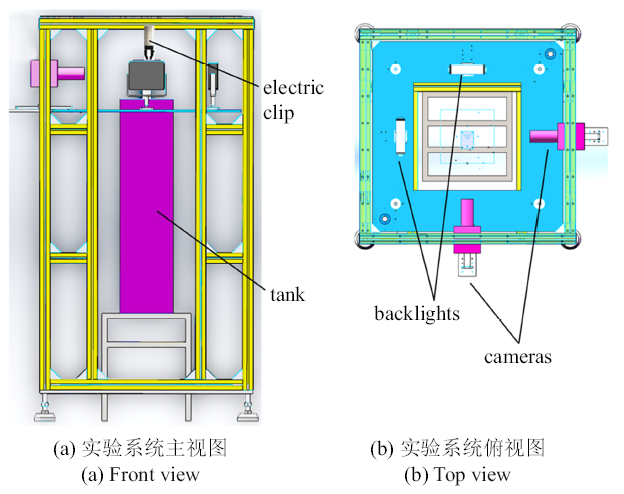Fig.1   Sketch of the experimental system

### 图2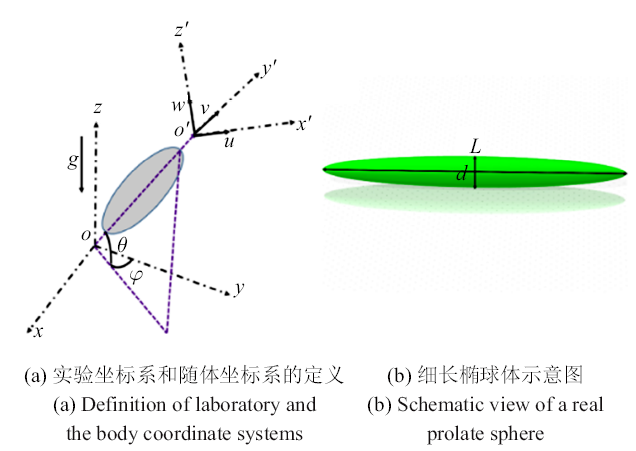Fig.2   Particle shape in experiments and the corresponding coordinate system

$Ar=\left( {\frac{\rho _{c} -\rho _{f} }{\rho _{f} }gD} \right)^{1/2}\frac{D}{\nu }$

Table 1  Characteristic properties of the 30 flow conditions considered in this study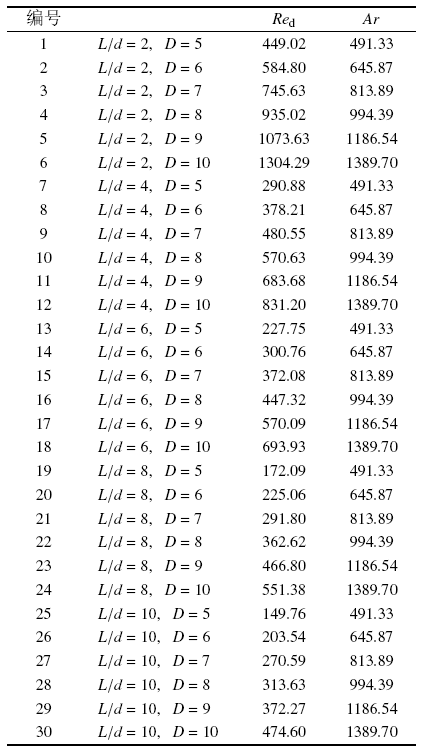### 图3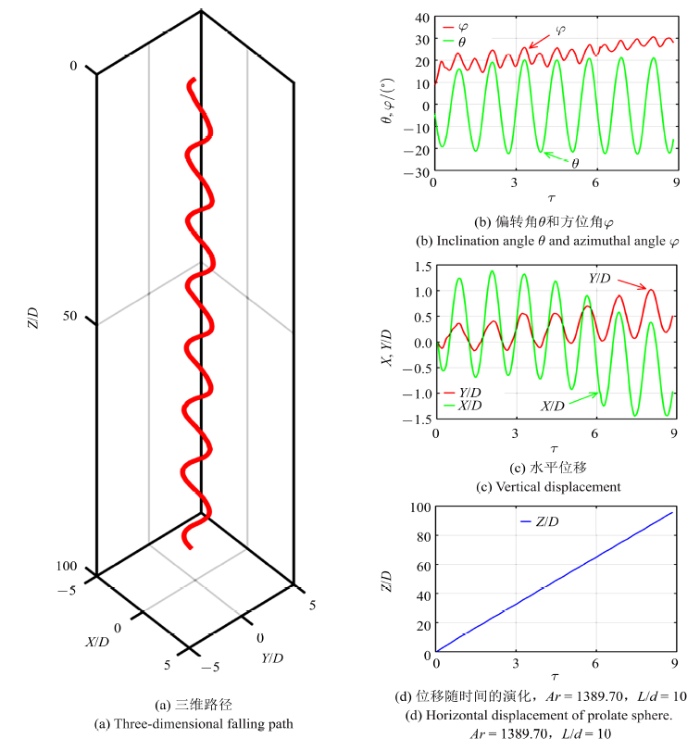Fig.3   Time histories of the falling behaviors of a prolate sphere

$\begin{eqnarray} &&\left[ \begin{array}{l} u \\ v \\ w \\ \end{array} \right]=R_\theta R_\varphi \left[\begin{array}{l} u_x \\ u_y \\ u_z \\ \end{array} \right] \\ && R_\theta =\left[\begin{array}{c@{\qquad }c@{\qquad }c} {\cos \theta } & 0 & {\sin \theta } \\ 0 & 1 & 0 \\ {-\sin \theta } & 0 & {\cos \theta } \\ \end{array}\right] \\ && R_\varphi =\left[ \begin{array}{c@{\qquad }c@{\qquad }c} {\cos \varphi } & {\sin \varphi } & 0 \\ {-\sin \varphi } & {\cos \varphi } & 0 \\ 0 & 0 & 1 \\ \end{array} \right] \end{eqnarray}$

### 图4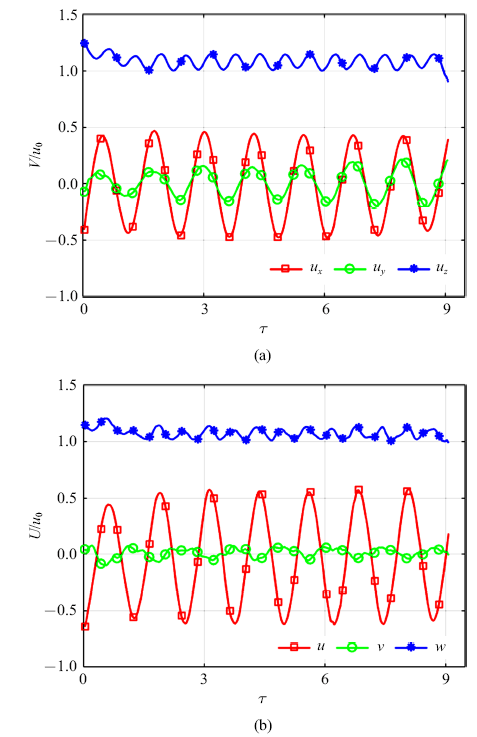Fig.4   Comparison of the velocity components (a) in the laboratory frame; and (b) in the system of rotating with the body (defined in Fig.2(a)). $Ar= 1389.70$, $L/d = 10$

## 2 实验结果与分析

### 图9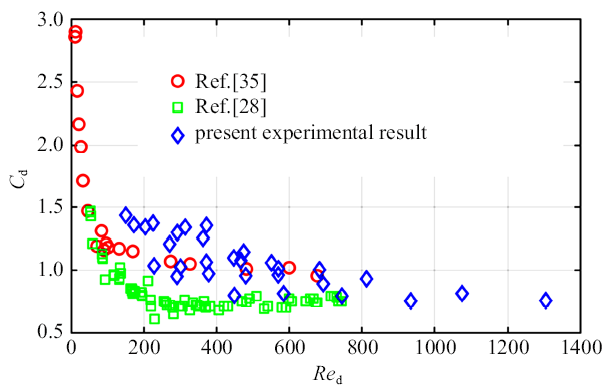Fig.9   Drag coefficient of the freely falling prolate spheres versus Reynolds number

### 图10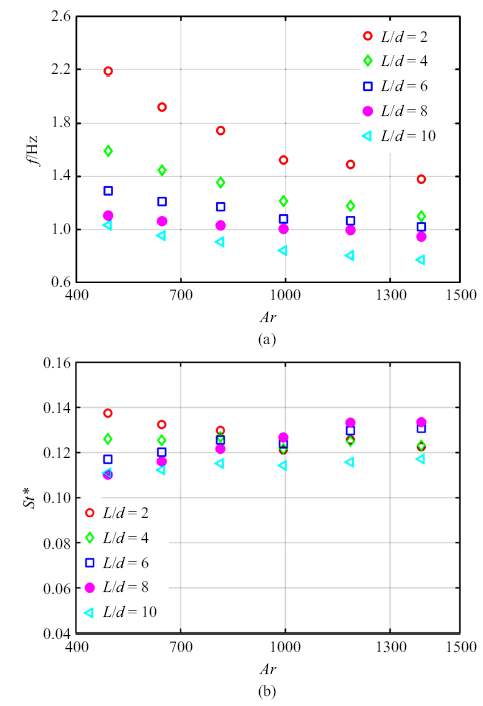Fig.10   Comparison of the oscillations frequency $f$ and the $St^{\ast }$ of the body inclination angle versus the $Ar$ for various elongation ratio $L/d$

### 图11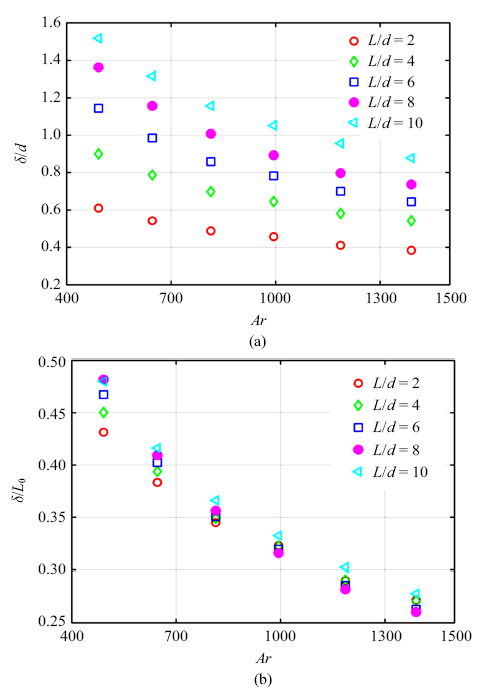Fig.11   Thickness of the unsteady boundary layer $\delta$ versus $Ar$ for various elongation ratios, normalized with the prolate sphere minor axis $d$ and $L_0 =\sqrt{dL}$

### 图12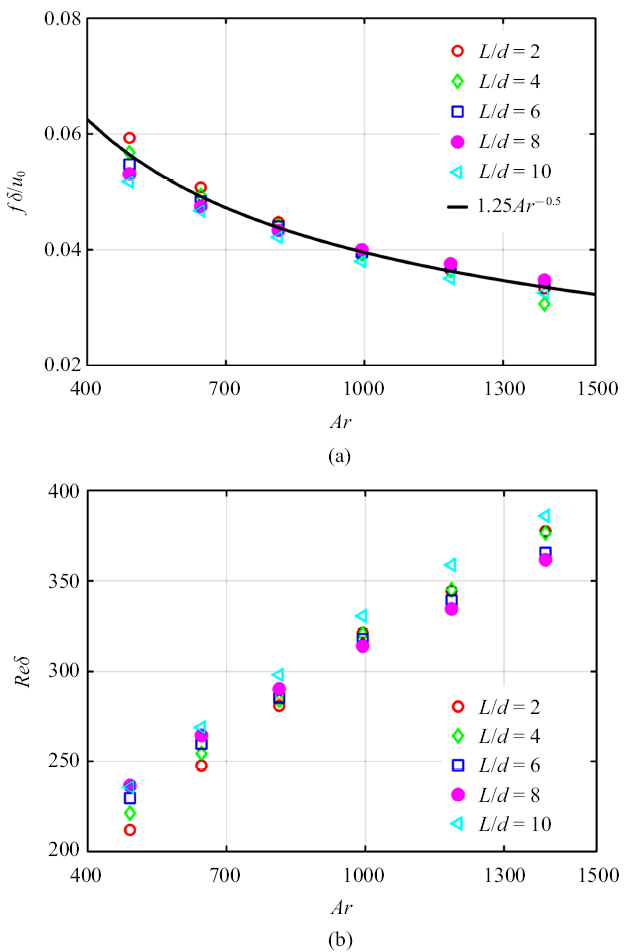Fig.12   Based on the thickness of the unsteady boundary. $St^{\ast}$ and Reynolds number versus $Ar$

### 图13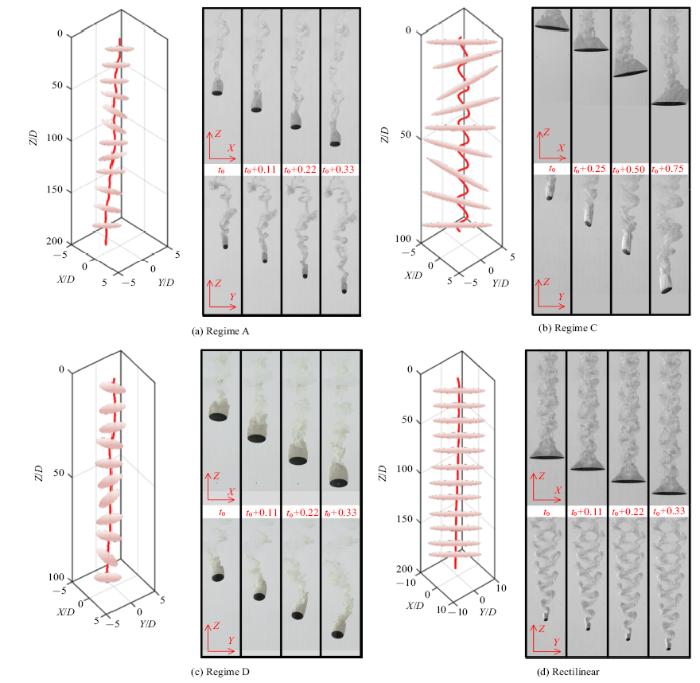Fig.13   Examples of the time of evolution of three-dimensional path and corresponding wake , the upper and lower parts of each wake diagram are the front view and the side view of the time, respectively, (a) for the low-amplitude irregular motion ($L/d = 2$, $Re_{d}$ = 449.02); (b) for the high-amplitude fluttering ($L/d$ = 10, $Re_{d}$ = 474.60), (c) for the highly nonlinear motion ($L/d$ = 2, $Re_{d}$ = 1304.29); and (d) for the rectilinear motion ($L/d$ = 10, $Re_{d}$ = 149.76)

## 3 结论

(1) 对于所研究的参数范围($2\leqslant L/d \leqslant 10$, $400\leqslant Ar\leqslant1400$), 根据偏转角以及水平速度的演化特点将路径分为5类: 小振幅不规则运动(A状态)、包括小振幅高频振荡运动(B状态)、大振幅低频振荡(C状态)、高度非线性运动(D状态)、直线运动. 这五类运动路径与物体形状以及体积有关.

(2) 在相同参数范围下本文研究的细长椭球体受到的阻力系数$C_{d}$明显大于Toupoint等 对于圆柱阻力系数的测量结果, 产生阻力增大的主要原因是本文研究的椭球体相比圆柱体在下落时的偏转角\lb 更大.

(3) 通过引入特征长度尺度$\delta =2\pi \sqrt {2\nu /\omega }$和特征速度尺度$u_0 =\left[ {\left( {\rho _{c} /\rho _{f} -1} \right)gd}\right]^{1/2}$, 发现$St^{\ast}= f\delta/u_{0}$和$Re_{d}=u_{0}\delta/\nu$取决于$Ar$变化而与$L/d$无关. 一方面说明物体的体积是很重要的参数而不再是具体的物体形状, 另一方面说明椭球体的尖端在控制运动物体附近的非定常边界层的扩展有很重要的作用, 这一点和细长圆柱体两端的作用类似.

(4) 通过对各种路径下尾涡的研究, 首先验证了Mehdi等 中细长椭球体会绕垂直轴旋转这一结论. 其次通过对不同细长椭球体尾迹的观察可以发现, 一方面随着雷诺数的增大, 尾迹会变得越来越不规则; 另一方面当$L/d \to 1$时, 细长椭球体的运动状态和球体的类似, $L/d \to 10$时, 细长椭球体的运动状态和细长圆柱($L/d$ = 10)的类似.

/

 〈〉